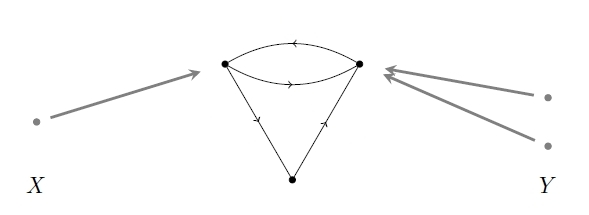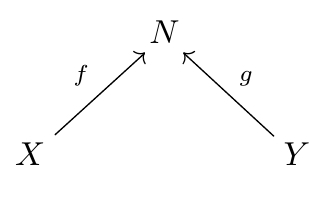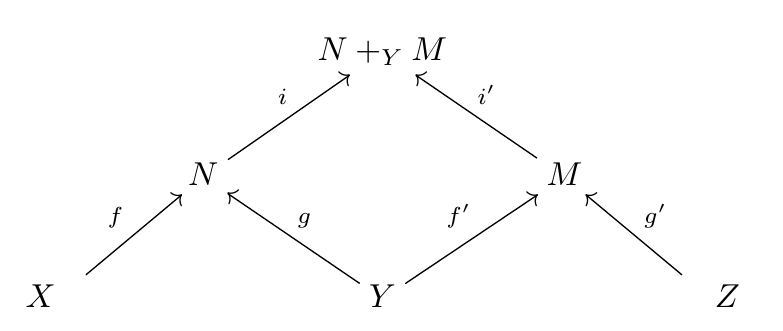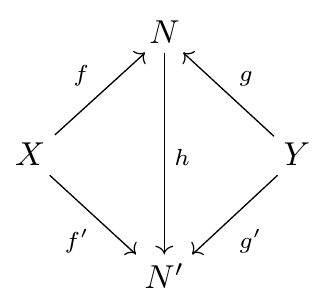## September 16, 2020

### Open Systems: A Double Categorical Perspective (Part 2)

#### Posted by John BaezBack to Kenny Courser’s thesis:

One thing Kenny does here is explain the flaws in a well-known framework for studying open systems: decorated cospans. Decorated cospans were developed by my student Brendan Fong. Since I was Brendan’s advisor at the time, a hefty helping of blame for not noticing the problems belongs to me! But luckily, Kenny doesn’t just point out the problems: he shows how to fix them. As a result, everything we’ve done with decorated cospans can be saved.

The main theorem on decorated cospans is correct; it’s just less useful than we’d hoped! The idea is to cook up categories where the morphisms are open systems. The objects of such a category could be something simple like finite sets, but morphisms from $X$ to $Y$ could be something more interesting, like ‘open graphs’:Here $X$ and $Y$ are mapped into a third set in the middle, but this set in the middle is the set of nodes of a graph. We say the set in the middle has been ‘decorated’ with a graph.

Here’s how the original theory of decorated cospans seeks to make this precise.

Fong’s Theorem. Suppose $\mathsf{A}$ is a category with finite colimits, and make $\mathsf{A}$ into a symmetric monoidal category with its coproduct as the tensor product. Suppose $F\colon (\mathsf{A},+) \to (\mathsf{Set},\times)$ is a symmetric lax monoidal functor. Define an F-decorated cospan to be a cospanin $\mathsf{A}$ together with an element of $F(N)$. Then there is a symmetric monoidal category with

• objects of $\mathsf{A}$ as objects,
• isomorphism classes of $F$-decorated cospans as morphisms.

I won’t go into many details, but let me say how to compose two decorated spans, and also how this ‘isomorphism class’ business works.

Given two decorated cospans we compose their underlying cospans in the usual way, via pushout:We get a cospan from $X$ to $Z$. To decorate this we need an element of $F(M +_Y N)$. So, we take the decorations we have on the cospans being composed, which together give an element of $F(N) \times F(M)$, and apply this composite map:

$F(N) \times F(M) \longrightarrow F(N+M) \longrightarrow F(N+_Y M)$

Here the first map, called the laxator, comes from the fact that $F$ is a lax monoidal functor, while the second comes from applying $F$ to the canonical map $N+M \to N+_Y M$.

Since composing cospans involves a pushout, which is defined via a universal property, the composite is only well-defined up to isomorphism. So, to get an actual category, we take isomorphism classes of decorated cospans as our morphisms.

Here an isomorphism of cospans is a commuting diagram like this:where $h$ is an isomorphism. If the first cospan here has a decoration $d \in F(N)$ and the second has a decoration $d' \in F(N')$, then we have an isomorphism of decorated cospans if $F(h)(d) = d'$.

So, that’s the idea. The theorem is true, and it works fine for some applications — but not so well for others, like the example of open graphs!

Why not? Well, let’s look at that example in detail. Given a finite set $N$, let’s define a graph on $N$ to be a finite set $E$ together with two functions $s, t \colon E \to N$. We call $N$ the set of nodes, $E$ the set of edges, and the functions $s$ and $t$ map each edge to its source and target, respectively. So, a graph on $N$ is a way of choosing a graph whose set of nodes is $N$.

We can try to apply the above theorem taking $\mathsf{A} = \mathsf{FinSet}$ and letting $F \colon \mathsf{FinSet} \to \mathsf{Set}$be the functor sending each finite set $N$ to the set of all graphs on $N$.

The first problem, which Brendan and I noticed right away, is that there’s not really a set of graphs on $N$. There’s a proper class! $E$ ranges over all possible finite sets, and there’s not a set of all finite sets.

This should have set alarm bells ringing right away. But we used a standard dodge. In fact there are two. One is to replace $\mathsf{FinSet}$ with an equivalent small category, and define a graph just as before but taking $N$ and $E$ to be objects in this equivalent category. Another is to invoke the axiom of universes. Either way, we get a set of graphs on each $N$.

Then Fong’s theorem applies, and we get a decorated cospan category with:

• ‘finite sets’ as objects,
• isomorphism classes of open graphs as morphisms.

Here I’m putting ‘finite sets’ in quotes because of the trickery I just mentioned, but it’s really not a big deal so I’ll stop now. An open graph has a finite set $N$ of nodes, a finite set $E$ of edges, maps $s,t \colon E \to N$ saying the source and target of each edge, and two maps $f \colon X \to N$ and $g \colon Y \to N$.

These last two maps are what make it an open graph going from $X$ to $Y$:Isomorphism classes of open graphs from $X$ to $Y$ are the morphisms from $X$ to $Y$ in our decorated cospan category.

But later, when Kenny was devising a bicategory of decorated cospans, we noticed a second problem. The concept of ‘isomorphic decorated cospan’ doesn’t behave well in this example: the concept of isomorphism is too narrowly defined!

Suppose you and I have isomorphic decorated cospans:In the example at hand, this means you have a graph on the finite set $N$ and I have a graph on the finite set $N'$. Call yours $d \in F(N)$ and mine $d' \in F(N')$.

We also have a bijection $h \colon N \to N'$ such that

$F(h)(d) = d'$

What does this mean? I haven’t specified the functor $F$ in detail so you’ll have to take my word for it, but it should be believable. It means that my graph is exactly like yours except that we replace the nodes of your graph, which are elements of $N$, by the elements of $N'$ that they correspond to. But this means the edges of my graph must be exactly the same as the edges of yours. It’s not good enough for our graphs to have isomorphic sets of edges: they need to be equal!.

For a more precise account of this, with pictures, read the introduction to Chapter 3 of Kenny’s thesis.

So, our decorated cospan category has ‘too many morphisms’. Two open graphs will necessarily define different morphisms if they have different sets of edges.

This set Kenny and I to work on a new formalism, structured cospans, that avoids this problem. Later Kenny and Christina Vasilakopoulou also figured out a way to fix the decorated cospan formalism. Kenny’s thesis explains all this, and also how structured cospans are related to the ‘new, improved’ decorated cospans.

But then something else happened! Christina Vasilakopoulou was a postdoc at U.C. Riverside while all this was going on. She and my grad student Joe Moeller wrote a paper on something called the monoidal Grothendieck construction, which plays a key role in relating structured cospans to the new decorated cospans. But the anonymous referee of their paper pointed out another problem with the old decorated cospans!

Briefly, the problem is that the functor $F \colon (\mathsf{FinSet},+) \to (\mathsf{Set},\times)$ that sends each $N$to the set of graphs having $N$ as their set of vertices cannot be made lax monoidal in the desired way. To make $F$ lax monoidal, we must pick a natural transformation called the laxator:

$\phi_{N,M} \colon F(N) \times F(M) \to F(N+M)$

I used this map earlier when explaining how to compose decorated cospans.

The idea seems straightforward enough: given a graph on $N$ and a graph on $M$ we get a graph on their disjoint union $N+M$. This is true, and there is a natural transformation $\phi_{N,M}$ that does this.

But the definition of lax monoidal functor demands that the laxator make a certain hexagon commute! And it does not!

I won’t draw this hexagon here; you can see it at the link or in the intro to Chapter 3 of Kenny’s thesis, where he explains this problem. The problem arises because when we have three sets of edges, say $E,E',E''$, we typically have

$(E + E') + E'' \ne E + (E' + E'')$

There is a sneaky way to partially get around this problem, which he also explains: define graphs using a category equivalent to $\mathsf{FinSet}$ where $+$ is strictly associative, not just up to isomorphism!

This is good enough to make $F$ lax monoidal. But Kenny noticed yet another problem: $F$ is still not symmetric lax monoidal. If you try to show it is, you wind up needing two graphs to be equal: one with $E+E'$ as its set of edges, and another with $E'+E$ as its set of edges. These aren’t equal! And at this point it seems we hit a dead end. While there’s a category equivalent to $\mathsf{FinSet}$ where $+$ is strictly associative, there’s no such category where $+$ is also strictly commutative.

In the end, by going through all these contortions, we can use a watered-down version of Fong’s theorem to get a category with open graphs as morphisms, and we can make it monoidal — but not symmetric monoidal.

It’s clear that something bad is going on here. We are not following the tao of mathematics. We keep wanting things to be equal, that should only be isomorphic. The problem is that we’re treating a graph on a set as extra structure on that set, when it’s actually extra stuff.

Structured cospans, and the new decorated cospans, are the solution! For example, the new decorated cospans let us use a category of graphs with a given set of nodes, instead of a mere set. Now $F(N)$ is a category rather than a set. This category lets us talk about isomorphic graphs with the same set of vertices, and all our problems evaporate.

• Part 1: an overview of Courser’s thesis and related papers.

• Part 2: problems with the original decorated cospans.

• Part 3: the new improved decorated cospans.

Posted at September 16, 2020 5:14 PM UTC

TrackBack URL for this Entry:   https://golem.ph.utexas.edu/cgi-bin/MT-3.0/dxy-tb.fcgi/3254